# Calculating the pH at equi.. A chemist titrates 70.0 ml. of a 04557 M carbonic acid...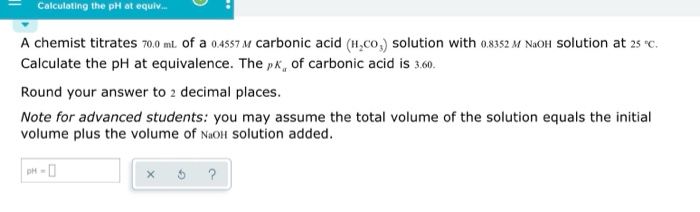Calculating the pH at equi.. A chemist titrates 70.0 ml. of a 04557 M carbonic acid (H,co,) solution with o8352 M NaOlH Solution at 2s c Calculate the pH at equivalence. The pk, of carbonic acid is 3.60 Round your answer to 2 decimal places. Note for advanced students: you may assume the total volume of the solution equals the initial volume plus the volume of NaoH Solution added. рH - D

use:

pKa = -log Ka

3.6 = -log Ka

Ka = 2.512*10^-4

find the volume of NaOH used to reach equivalence point

M(H2CO3)*V(H2CO3) =M(NaOH)*V(NaOH)

0.4557 M *70.0 mL = 0.8352M *V(NaOH)

V(NaOH) = 38.1932 mL

Given:

M(H2CO3) = 0.4557 M

V(H2CO3) = 70 mL

M(NaOH) = 0.8352 M

V(NaOH) = 38.1932 mL

mol(H2CO3) = M(H2CO3) * V(H2CO3)

mol(H2CO3) = 0.4557 M * 70 mL = 31.899 mmol

mol(NaOH) = M(NaOH) * V(NaOH)

mol(NaOH) = 0.8352 M * 38.1932 mL = 31.899 mmol

We have:

mol(H2CO3) = 31.899 mmol

mol(NaOH) = 31.899 mmol

31.899 mmol of both will react to form HCO3- and H2O

HCO3- here is strong base

HCO3- formed = 31.899 mmol

Volume of Solution = 70 + 38.1932 = 108.1932 mL

Kb of HCO3- = Kw/Ka = 1*10^-14/2.512*10^-4 = 3.981*10^-11

concentration ofHCO3-,c = 31.899 mmol/108.1932 mL = 0.2948M

HCO3- dissociates as

HCO3- + H2O -----> H2CO3 + OH-

0.2948 0 0

0.2948-x x x

Kb = [H2CO3][OH-]/[HCO3-]

Kb = x*x/(c-x)

Assuming x can be ignored as compared to c

So, above expression becomes

Kb = x*x/(c)

so, x = sqrt (Kb*c)

x = sqrt ((3.981*10^-11)*0.2948) = 3.426*10^-6

since c is much greater than x, our assumption is correct

so, x = 3.426*10^-6 M

[OH-] = x = 3.426*10^-6 M

use:

pOH = -log [OH-]

= -log (3.426*10^-6)

= 5.4652

use:

PH = 14 - pOH

= 14 - 5.4652

= 8.5348

#### Earn Coin

Coins can be redeemed for fabulous gifts.

Similar Homework Help Questions
• ### A chemist titrates 160.0 mL of a 0.3337 M carbonic acid (H2CO3) solution with 0.4095 M...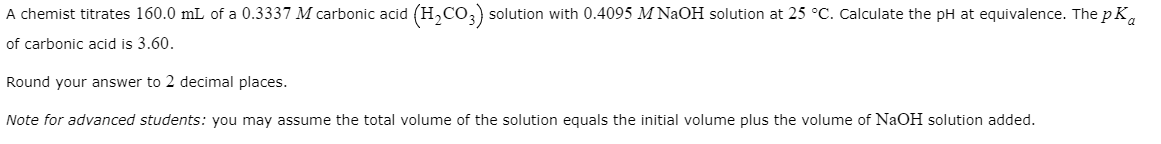A chemist titrates 160.0 mL of a 0.3337 M carbonic acid (H2CO3) solution with 0.4095 M NaOH solution at 25 °C. Calculate the pH at equivalence. The pK, of carbonic acid is 3.60. Round your answer to 2 decimal places. Note for advanced students: you may assume the total volume of the solution equals the initial volume plus the volume of NaOH solution added.

• ### A chemist titrates 90.0 mL of a 0.5705 M carbonic acid (H,CO,) solution with 0.6274 M...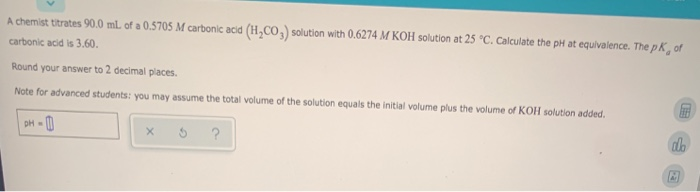A chemist titrates 90.0 mL of a 0.5705 M carbonic acid (H,CO,) solution with 0.6274 M KOH solution at 25 °C. Calculate the pH at equivalence. The pk of carbonic acid is 3.60. Round your answer to 2 decimal places. Note for advanced students: you may assume the total volume of the solution equals the initial volume plus the volume of KOH solution added. DH-

• ### A chemist titrates 130.0 mL of a 0.5266 M carbonic acid (H.CO) solution with 0.5751 M...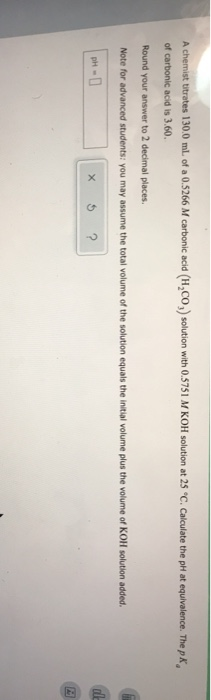A chemist titrates 130.0 mL of a 0.5266 M carbonic acid (H.CO) solution with 0.5751 M KOH solution at 25 °C. Calculate the pH at equivalence. The pk of carbonic acid is 3.60. Round your answer to 2 decimal places. Note for advanced students: you may assume the total volume of the solution equals the initial volume plus the volume of KOH solution added. en de ?

• ### - Calculating the pH at equivalence of a titration A chemist titrates 180.0 ml of a...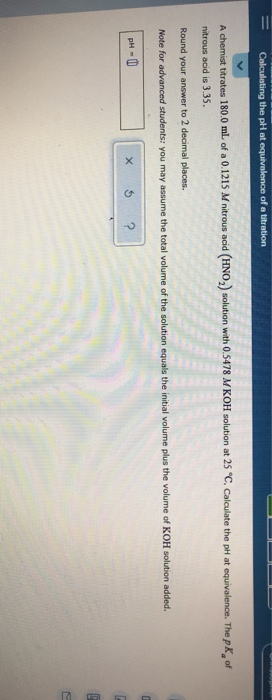- Calculating the pH at equivalence of a titration A chemist titrates 180.0 ml of a 0.1215 M nitrous acid (HNO) solution with 0.5478 M KOH solution at 25 "C. Calculate the pH at equivalence. The pk of nitrous acid is 3.35. Round your answer to 2 decimal places. Note for advanced students: you may assume the total volume of the solution equals the initial volume plus the volume of KOH solution added. PHU X 5 ?

• ### = O ACIDS AND BASES Calculating the pH at equivalence of a titration A chemist titrates...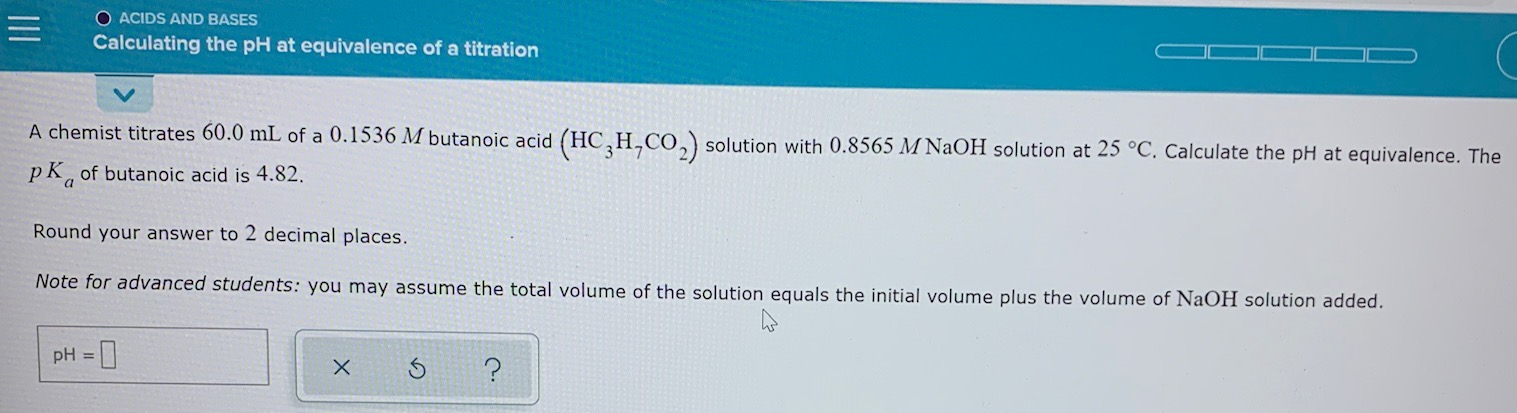= O ACIDS AND BASES Calculating the pH at equivalence of a titration A chemist titrates 60.0 mL of a 0.1536 M butanoic acid (HC,H,CO2) solution with 0.8565 M NaOH solution at 25 °C. Calculate the pH at equivalence. The pk, of butanoic acid is 4.82. Round your answer to 2 decimal places. Note for advanced students: you may assume the total volume of the solution equals the initial volume plus the volume of NaOH solution added. pH = x...

• ### A chemist titrates 190.0 mL of a 0.4427 Macetic acid (HCH,CO, solution with 0.2382 M NaOH...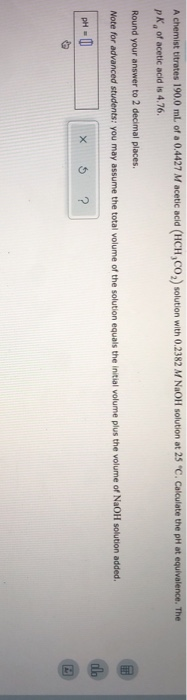A chemist titrates 190.0 mL of a 0.4427 Macetic acid (HCH,CO, solution with 0.2382 M NaOH solution at 25 "C. Calculate the pH at equivalence. The pk of acetic acid is 4.76. Round your answer to 2 decimal places. Note for advanced students: you may assume the total volume of the solution equals the initial volume plus the volume of NaOH solution added. pH = 0 x 5 ?

• ### Calculating the pH at equivalence of a titration A chemist titrates 120.0 mL of a 0.7513...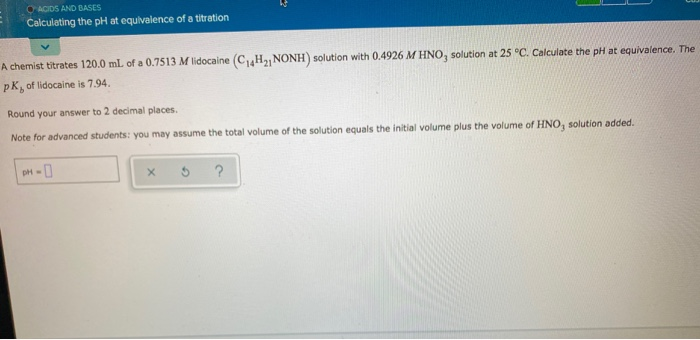Calculating the pH at equivalence of a titration A chemist titrates 120.0 mL of a 0.7513 M lidocaine (C4H2, NONH) solution with 0.4926 M HNO, solution at 25°C. Calculate the pH at equivalence. The PK, of lidocaine is 7.94 Round your answer to 2 decimal places Note for advanced students: you may assume the total volume of the solution equals the initial volume plus the volume of HNO, solution added. DH-

• ### A chemist titrates 200.0 mL of a 0.7681 M hydrocyanic acid (HCN) solution with 0.5271 M...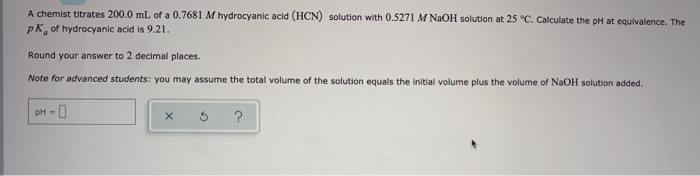A chemist titrates 200.0 mL of a 0.7681 M hydrocyanic acid (HCN) solution with 0.5271 M NaOH solution at 25 °C. Calculate the pH at equivalence. The pk of hydrocyanic acid is 9.21. Round your answer to 2 decimal places. Note for advanced students: you may assume the total volume of the solution equals the initial volume plus the volume of NaOH solution added. pH- х ?

• ### ACIDS AND BASES Calculating the pH at equivalence of a titration A chemist titrates 200.0 mL...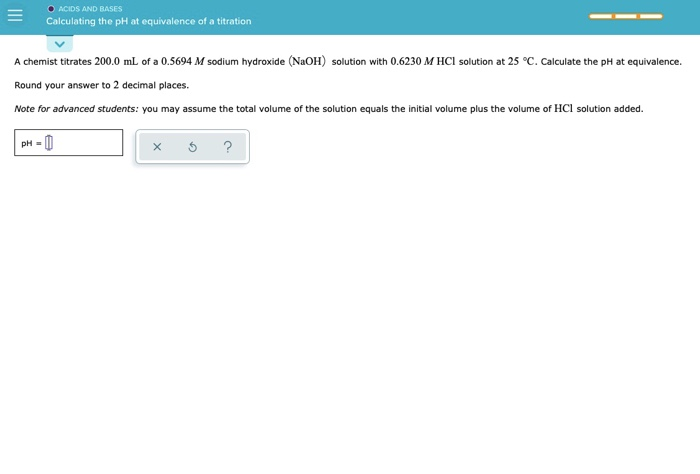ACIDS AND BASES Calculating the pH at equivalence of a titration A chemist titrates 200.0 mL of a 0.5694 M sodium hydroxide (NaOH) solution with 0.6230 M HCl solution at 25 °C. Calculate the pH at equivalence. Round your answer to 2 decimal places. Note for advanced students: you may assume the total volume of the solution equals the initial volume plus the volume of HCl solution added. PH-0 5 ?

• ### ACIDS AND BASES Calculating the pH at equivalence of a titration A chemist titrates 130.0 mL...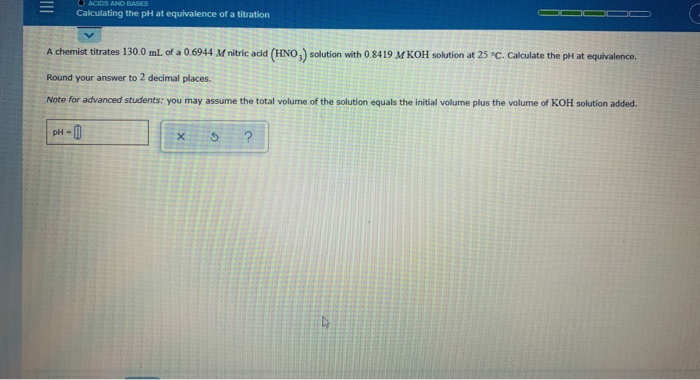ACIDS AND BASES Calculating the pH at equivalence of a titration A chemist titrates 130.0 mL of a 0.6944 M nitric acid (HNO.) solution with 0.8419 M KOH solution at 25 °C. Calculate the pH at equivalence Round your answer to 2 decimal places. Note for advanced students: you may assume the total volume of the solution equals the initial volume plus the volume of KOH solution added. pH-0 X 5 ?